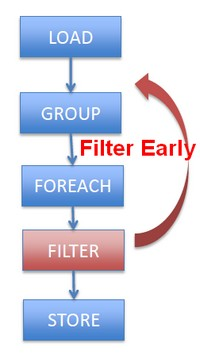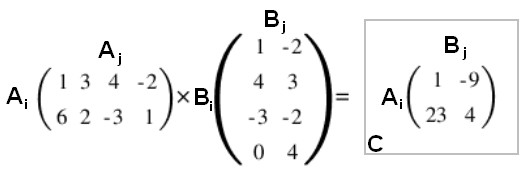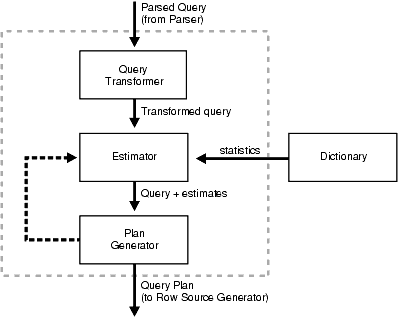# (Function|Operator) - Commutative property (Order doesn’t matter)

Commutative is an algebraic law.

By commutative property , order in the operand doesn’t matter.

## Example

 Addition a + b = b + a Multiplication x . y = y . x

## Documentation / Reference

Recommended Pages(Function|Operator) - Algebraic (Laws|properties) - Axioms

Property of function and operator Continuous SmoothAlgebraic Optimization

Same as: Algebraic Laws: (+) identity: x+0 = x (/) identity: x/1 = x () distributes: (nx+ny) = n(x+y) () commutes: xy = yx Apply rules 1,3,4,2 and we get Two operations instead of five,...Cryptography - Algorithm (called a Cipher)

A cipher is an algorithm that encrypt a plain text message into a ciphertext. Cipher algorithm can be categorized by the key that they used as parameters. No key symmetric one (using one key) ...Data Warehouse

A data warehouse is a large central data repository of current, history and summarised data coming from operational and external sources used primarily for analysis. s is large historical databases for...Linear Algebra - Matrix Matrix (Multiplication)

Matrix Matrix (Multiplication) definition . Two matrices may be multiplied when they are conformable: ie the number of columns in the first matrix is equal to the number of rows in the second matrix....Number - Multiplication (Product)

Multiplication is denoted by: the cross symbol × (\times in Latex), by a point · or by the absence of symbol It's a binary function. Multiplication is: Associative: By: Rafael...Parallel Programming - (Function|Operation)

A function or an operator has to be: commutative and associative in order to be computed correctly in parallel. A properly constructed operation is inherently parallelism, so long as the function(s)...SQL Engine - Query Optimizer (Query Optimization)

in a SQL Engine. A SQL statement can be executed in many different ways, such as: full table scans, index scans, nested loops, hash joins. The query optimizer determines the most efficient...